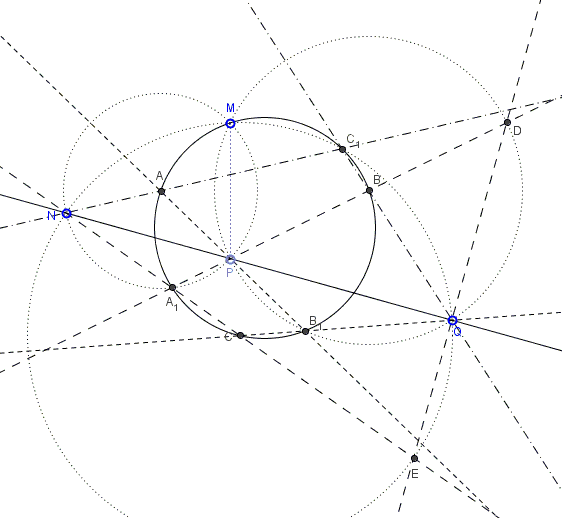# Seven Concyclic Points

The problem below was posted posted at the CutTheKnotMath facebook page by Cõ Gẫng Lên:

Points $N, P, Q$ are collinear. For a fourth point $M,$ consider circumcircles, $(A)$ of $\Delta MNP$, $(B)$ of $\Delta MPQ$, $(C)$ of $\Delta NPQ.$ In addition, let $A_{1}$ be the intersection of $CN$ and $BP,$ $B_{1}$ the intersection of $AP$ and $CQ,$ and $C_{1}$ the intersection of $AN$ and $BQ.$Prove that points $M, A, B, C, A_{1}, B_{1}, C_{1}$ are concyclic.

(The applet below illustrates the problem.)

SolutionPoints $N, P, Q$ are collinear. For a fourth point $M,$ consider circumcircles, $(A)$ of $\Delta MNP$, $(B)$ of $\Delta MPQ$, $(C)$ of $\Delta NPQ.$ In addition, let $A_{1}$ be the intersection of $CN$ and $BP,$ $B_{1}$ the intersection of $AP$ and $CQ,$ and $C_{1}$ the intersection of $AN$ and $BQ.$Prove that points $M, A, B, C, A_{1}, B_{1}, C_{1}$ are concyclic.

### Solution

We already proved that points $M,A,B,C$ are concyclic. This followed from the identity $\angle AMB+\angle ACB=180^{\circ}$ which was a consequence of the fact that triangles $AMN$ and $BMQ$ are obtained from each other by a rotation around $M$ with subsequent homothety with $M$ as center. The combined transformation maps line $AN$ onto $BQ$ so that $\angle AC_{1}B$ is exactly the angle of rotation, implying $\angle AC_{1}B=\angle AMB$ which places $C_1$ on the circumcircle $MABC.$

... to be continued ...